# PSEB 10th Class Maths Solutions Chapter 2 Polynomials Ex 2.3

Punjab State Board PSEB 10th Class Maths Book Solutions Chapter 2 Polynomials Ex 2.3 Textbook Exercise Questions and Answers.

## PSEB Solutions for Class 10 Maths Chapter 2 Polynomials Ex 2.3

Question 1.
Apply the division algorithm to find the quotient and remainder on dividing p (x) by g (x) as given below:
(i) p (x) = x3 – 3x2 + 5x – 3, g (x) = x2 – 2 (Pb. 2018 Set I, II, III)
(ii) p (x) = x4 – 3x2 + 4x + 5, g(x) = x2 + 1 – x
(iii) p (x) = x4 – 5x + 6, g (x) = 2 – x2 [Pb. 2017 Set-B]
Solution:
(i) Given that p (x) = x3 – 3x2 + 5x – 3 and g (x) = x2 – 2,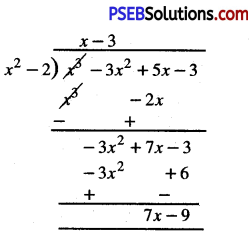By division algorithm,
x3 – 3x2 + 5x – 3 = (x – 3) (x2 – 2) + (7x – 9)
Hence, quotient = x – 3 and remainder = 7x – 9(ii) Given that p (x) = x4 – 3x2 + 4x + 5
or p (x) = x4 + 0x3 – 3x2 + 4x + 5
and g (x) = x2 + 1 – x
or g (x) = x2 – x + 1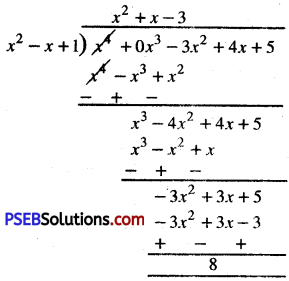By Division Algorithm,
x4 – 3x2 + 4x + 5 = (x2 + x – 3) (x2 – x + 1) + 8
Hence, Quotient = x2 + x – 3 and remainder = 8(iii) Given that p (x) = x4 – 5x + 6
or p (x) = x4 + 0x3 + 0x2 – 5x + 6
and g (x) = 2 – x2
or g (x) = – x2 + 2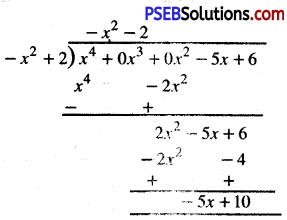By division algorithtm,
x4 – 5x + 6 = (- x2 – 2) (- x2 + 2) + (- 5x + 10)
Hence, quotient = -x2 – 2.
remainder = – 5x + 10

Question 2.
Check whether the first polynomial is a factor of the second polynomial by applying the division algorithm:
(i) t2 – 3, 2t4 + 3t3 – 2t2 – 9t – 12
(ii) x2 + 3x + 1, 3x4 + 5x3 – 7x2 + 2x + 2
(iii) x3 – 3x + 1, x5 – 4x3 + x2 + 3x + 1
Solution: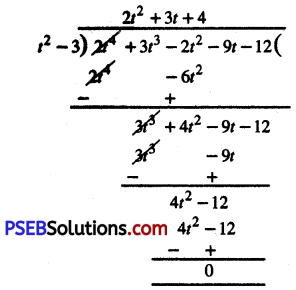∵ remainder is zero
∵ By division algorithm,
t2 – 3 is factor of 2t4 + 3t3 – 2t2 – 9t – 12

(ii)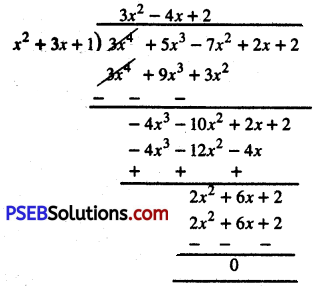∵ remainder is zero
∴ By division algorithm, x2 + 3x + 1 is a factor of 3x4 + 5x3 – 7x2 + 2x + 2

(iii)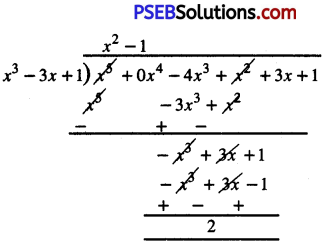∵ remainder is not zero.
∴ By division algorithm, x3 – 3x + 1 is not a factor of x5 – 4x3 + x2 + 3x + 1.Question 3.
Obtain all other zeroes of 3x4 + 6x3 – 2x2 – 10x – 5, if two of its zeroes are $$\sqrt{\frac{5}{3}}$$ and – $$\sqrt{\frac{5}{3}}$$.
Solution:
Given that two zeroes are $$\sqrt{\frac{5}{3}}$$ and –$$\sqrt{\frac{5}{3}}$$
∴ (x – $$\sqrt{\frac{5}{3}}$$) [x – (-$$\sqrt{\frac{5}{3}}$$)] are factors of given polynomial or
(x – $$\sqrt{\frac{5}{3}}$$) (x + $$\sqrt{\frac{5}{3}}$$) are factors of given polynomial.
or x2 – $$\frac{5}{3}$$ is a factor of given polynomial.
Now, apply division algorithm to given polynomial and x2 – $$\frac{5}{3}$$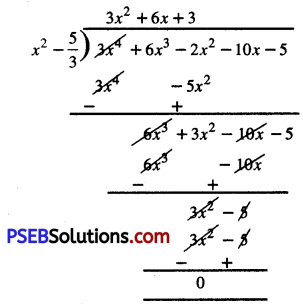∴ 3x4 + 6x3 – 2x2 – 10x – 5
= (x2 – $$\frac{5}{3}$$) (3x2 + 6x + 3)
= (x2 – $$\frac{5}{3}$$) (3) [x2 + 2x + 1]
= 3 (x2 – $$\frac{5}{3}$$) [x2 + 2x + 1]
[S = 2, P = 1]
= 3 (x2 – $$\frac{5}{3}$$) [x(x + 1) + 1 (x + 1)]
= 3 (x2 – $$\frac{5}{3}$$) (x + 1) (x + 1)
Now, other zeroes of polynomials are given by
x + 1 = 0 ; x = -1 or
x + 1 = 0 ; x = -1
The zeroes of the given fourth degree polynomial are :
$$\sqrt{\frac{5}{3}}$$, –$$\sqrt{\frac{5}{3}}$$, -1, -1.Question 4.
On dividing x3 – 3x2 + x + 2 by a polynomial g(x), the quotient and remainder were x – 2 and – 2x + 4 respectively find g(x).
Solution.
Compare given data with division algorithm, we have
p(x)= g (x). q(x) + r (x) or
p(x) – r(x) = g(x).q(x)
or g(x) . q (x) = p(x) – r(x)
or g(x) = $$\frac{p(x)-r(x)}{q(x)}$$
By putting various values, we get:
g(x) = $$\frac{\left(x^{3}-3 x^{2}+x+2\right)-(-2 x+4)}{x-2}$$

g(x) = $$\frac{x^{3}-3 x^{2}+x+2+2 x-4}{x-2}$$

g(x) = $$\frac{x^{3}-3 x^{2}+3 x-2}{x-2}$$ …………….(1)

Now,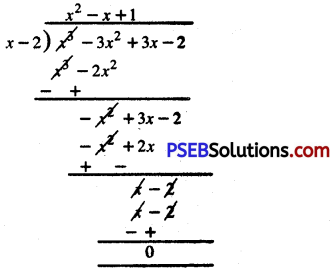∴ $$\frac{x^{3}-3 x^{2}+3 x-2}{x-2}$$ = x2 – x + 1
From (1) and (2), we get:
g(x) = x2 – x + 1Question 5.
Give examples of polynomials p(x), g(x), q(x) and r(x), which satisfy the dhriskm algorithm and
(i) deg p (x) = deg q (x)
(ii) deg r (x) = 0
(iii) deg q (x) = deg r (x)
Solution:
(i) Let p(x) = 5x2 – 5x +10; g(x) = 5 q(x) = x2 – x + 2; r(x) = 0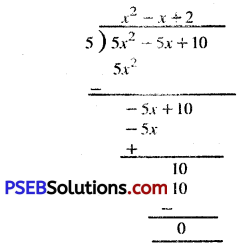∴ By division algorithm,
5x2 – 5x + 10 = 5(x2 – x + 2) + 0
or p(x) = g(x) q(x) + r(x)
Also, deg p(x) = deg q(x) = 2

(ii) Let p(x) = 7x3 – 42x + 53
g(x) = x3 – 6x + 7
q(x) = 7; r(x) = 4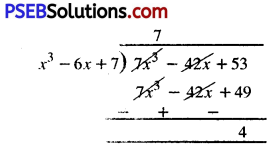∴ By division algorithm,
7x3 -42x + 53 = 7(x3 – 6x+ 7)+ 4
or p(x) = q(x) g(x) + r(x)
Also, deg q(x) = 0 = deg r(x)(iii) Let p(x) = 4x3 + x2 + 3x + 6;
g(x) = x2 + 3x + 1;
q(x) = 4x – 11;
r(x) = 32x + 17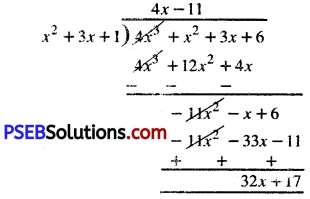∴ By division algorithm,
4x3 + x2 + 3x + 6 = (4x – 11) (x2 + 3x + 1) + (32x + 17)
or p(x) = q(x) . g(x) + r(x)
Also, deg q(x) = deg r(x)